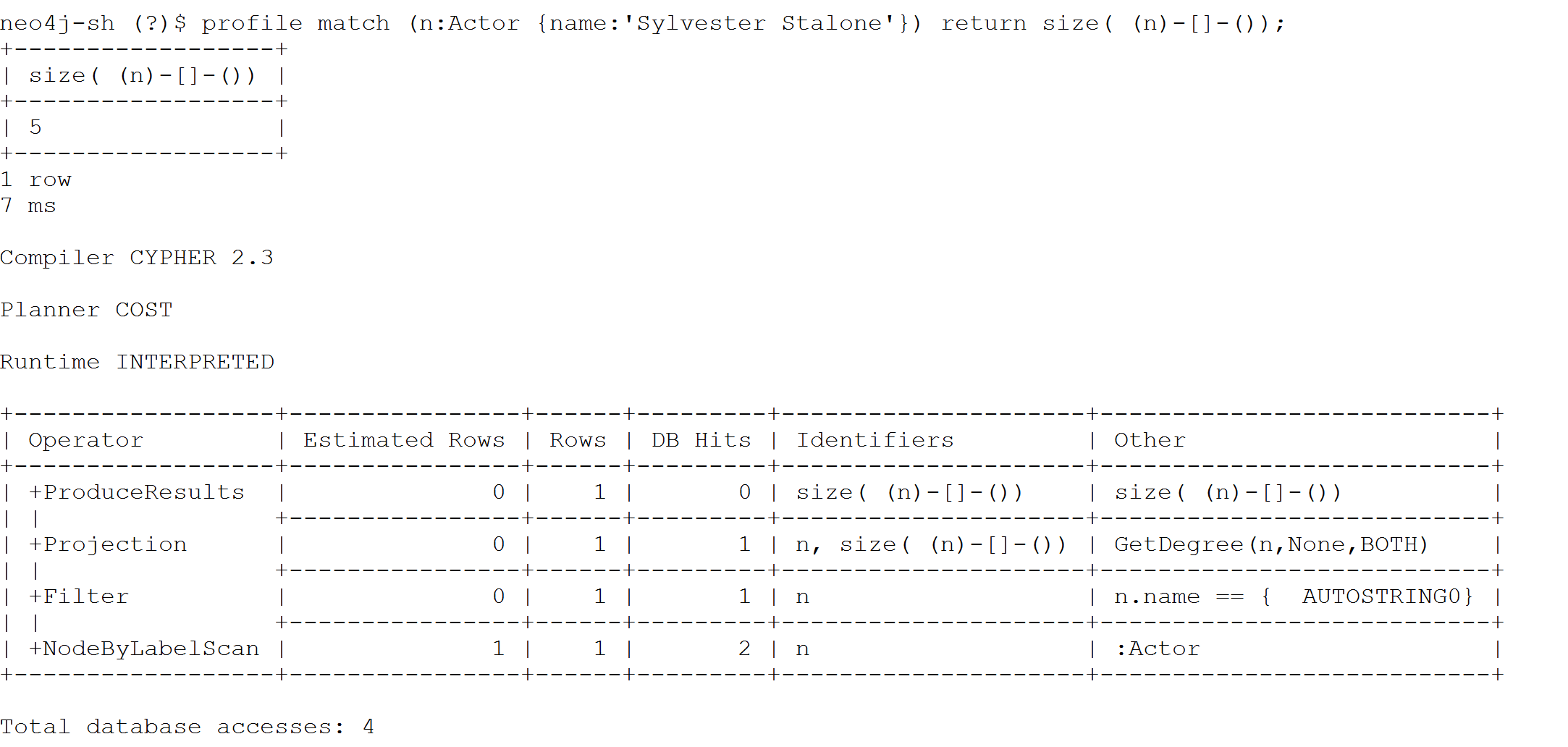# How do I improve the performance of counting number of relationships on a node

Using Cypher one could count number of relationships in the following manner

``MATCH (n:Actor {name:'Sylvester Stallone'})-->() RETURN count(*);``

Which will report the number of incoming/outgoing relationships for the `Actor` named Sylvester Stallone.

Using `bin/neo4j-shell` and running a profile on the query will produce the following outputThe Cypher can be rewritten to

``````MATCH (n:Actor {name:'Sylvester Stallone'})
RETURN size((n)-->())``````

And to which the profile is:From the profile above you will see a reference to `GetDegree(n,None,BOTH)` which occurs as a result of the usage of `size( (n)-[]-());` Because of this we do not actually perform a traversal of relationships from `(n)` but rather consult the degree value stored with the given node.

In the above `GetDegree()` expression, the 2nd parameter refers to the relationship name, and the 3rd parameter refers to the direction of the relationship.

For example the Cypher of

``````MATCH (n:Actor {name:'Sylvester Stallone'})
RETURN size( (n)-[:ACTED_IN]->())``````

would result in the following profile:and thus a `GetDegree(n,Some(ACTED_IN),OUT)`